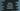# Python program to print numbers with comma as thousand separators## Introduction :

Let’s say your frontend is showing the same value that backend sends and if your python backend needs to send numbers with comma as the thousand separators, it is actually pretty simple. For example, if the input is 1000, it should convert it to 1,000 and if it is 100000, it should convert it to 100,000.

In this post, we will learn how to add thousand separator to a number.

## Method 1 :

``````def getThousandSeparator(num):
return '{:,}'.format(num)

print(getThousandSeparator(int(input("Enter a number : "))))``````

We are taking the user input as an integer and getThousandSeparator converts it to comma separated value. format with {:,} is used for the conversion. format was introduced in python 2.7. So, it will work only for python 2.7 and above.

Sample output :

``````Enter a number : 1234
1,234

Enter a number : 1000
1,000

Enter a number : 100
100

Enter a number : 123456789
123,456,789``````

If you are using python 3.7 or above, you can also write it like below :

``````def getThousandSeparator(num):
return '{value:,}'.format(value=num)

print(getThousandSeparator(int(input("Enter a number : "))))``````